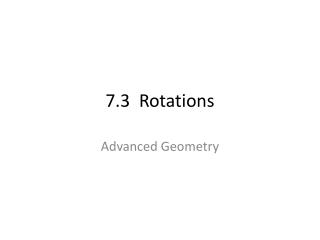Download Presentation7.3 Rotations

# 7.3 Rotations - PowerPoint PPT PresentationDownload Presentation## 7.3 Rotations

- - - - - - - - - - - - - - - - - - - - - - - - - - - E N D - - - - - - - - - - - - - - - - - - - - - - - - - - -
##### Presentation Transcript

2. ROTATIONS A rotation is a transformation in which a figure is turned about a fixed point. The fixed point is the center of rotation.

3. Angle of Rotation Rays drawn from the center of rotation to a point and its image form an angle called the angle of rotation.

4. Let’s Draw a Rotation First draw a triangle ABC and a point outside the triangle called P. We are going to draw a rotation of 120 degrees counterclockwise about P.

5. Next… Draw a segment connecting vertex A and the center of rotation point P. We will need to know the measurement of this segment later…might want to find that now.

6. Next…. Use a protractor to measure a 120 degree angle counterclockwise and draw a ray.

7. Next…. We want to cut that ray down so that it is congruent to segment PA. Name the endpoint A’. You could also do this with a compass

8. Next…. Repeat the steps for points B and C.

9. Example A quadrilateral has vertices P(3,-1), Q(4,0), R(4,3), and S(2,4). Rotate PQRS 180 degrees counterclockwise about the origin and name the coordinates of the new vertices.

10. Example Triangle RST has coordinates R(-2,3), S(0,4), and T(3,1). If triangle RST is rotated 90 degrees clockwise about the origin. What are the coordinates of the new vertices?

11. Rotation Tricks Rotation of 90° clockwise or ___° counterclockwise about the origin can be described as (x, y)  (y, -x)

12. Rotation Tricks Rotation of 270° clockwise or ___° counterclockwise about the origin can be described as (x, y)  (-y, x)

13. Rotation Tricks Rotation of 180°about the origin can be described as (x, y)  (-x, -y)

14. Example A quadrilateral has vertices A(-2, 0), B(-3, 2), C(-2, 4), and D(-1, 2). Give the vertices of the image after the described rotations. • 180° about the origin • 90° clockwise about the origin • 270° clockwise about the origin

15. Example A triangle has vertices F(-3, 3), G(1, 3), and H(1, 1). Give the vertices of the image after the described rotations. • 180° about the origin • 90° clockwise about the origin • 270° clockwise about the origin

16. Theorem 7.3 If lines k and m intersect at point P, then a reflection in k followed by a reflection in m is a rotation about point P. The angle of rotation is 2x°, where x° is the measure of the acute or right angle formed by k and m.

17. Example

18. Example If m and n intersect at Q to form a 30° angle. If a pentagon is reflected in m, then in n about point Q, what is the angle of rotation of the pentagon?

19. Rotational Symmetry A figure in the plane has rotational symmetry if the figure can be mapped onto itself by a rotation of 180° or less.

20. Rotational Symmetry Which figures have rotational symmetry? For those that do, describe the rotations that map the figure onto itself. 1. 2.

21. Examples Work on these problems from the book… Pg 416 #1-3, 6-12